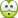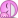# Damped and undamped oscillations (integration?)

## Homework Statement

Hi everybody! I'm doing a problem about oscillations, and I must admit that a few things are still unclear to me about that subject. Can someone maybe help me?

a) A onedimensional masspoint m is oscillating under the influence of the force $$F(x) = -c⋅x (c > 0).$$ What is the Newton's equation of motion for the system and integrate it with an exponential approach $$x(t) ∼ e^{λt}.$$ Find the integration constant for the initial conditions $$x(t = 0) = x_0$$ and $$\dot{x}(t = 0) = \dot{x_0}.$$
b) A friction force $$-ϒ \dot{x} (ϒ > 0)$$ is added to the system of a), that means a total force $$F(x,\dot{x}) = -cx - ϒ\dot{x}$$ is acting upon the masspoint m. Similarly to question a), find the path $$x(t)$$ and distinguish it between the cases:
$$0 < \frac{ϒ}{m} < 2ω_0 : \mbox{underdamping}$$
$$\frac{ϒ}{m} = 2ω_0 : \mbox{critical damping}$$
$$0 < 2ω_0 < \frac{ϒ}{m} : \mbox{overdamping}$$

## The Attempt at a Solution

a) So first I set up my equation of motion:

$$m \cdot \ddot{x} = -c \cdot x ⇔ m \cdot \ddot{x} + c \cdot x = 0 ⇔ \ddot{x} + \frac{c}{m} x = 0 \\ \mbox{Let } ω_0 = \sqrt{\frac{c}{m}} ⇒ \boldsymbol{\ddot{x} + ω_0^2 x = 0}$$
Then I rewrite the equation in the exponential form and solve for λ:
$$x(t) = α \cdot e^{λt} ⇒ α \cdot λ^2 \cdot e^{λt} + α \cdot ω_0^2 \cdot e^{λt} = 0 ⇔ λ^2 + ω_0^2 = 0 \\ ⇒ \boldsymbol{λ = ± i \cdot ω_0} ⇒ x(t) = α \cdot e^{i ω_0 t} + α^{*} \cdot e^{-i ω_0 t}$$
Then do I have to integrate the equation of motion by substituting x(t) by that expression? Why would we want to do that anyway? To get the velocity? It's the first time I'm ever asked to integrate an equation of motion, and there is no explanation whatsoever with the problem about why should one do that. Anyway I gave it a go:

$$\int -α \cdot ω_0^2 \cdot e^{i ω_0 t} + α \cdot ω_0^2 \cdot e^{-i ω_0 t} dt = -α \cdot ω_0^2 \int e^{i ω_0 t} dt+ α \cdot ω_0^2 \int e^{-i ω_0 t} dt \\ = i \cdot α \cdot ω_0 (e^{i ω_0 t} + \frac{1}{e^{-i ω_0 t}}) + C$$

Ehem...Not sure what is that, if it's correct and what to do from there!I do not know what α is, and all those imaginary units kind of scare me! Some help/hint here would be very appreciated. :)

Julien.

BvU
Homework Helper
Hi Julien,
Then do I have to integrate the equation of motion
You've done that by solving ! You assumed a solution of the given form and found it satisfies the differential equation if ##\lambda = i\omega## and also if ##\lambda = -i\omega##. Since the equation is linear, ##x(t) = A e^{i\omega t} + B e^{-i\omega t}## also satisfies the equation of motion

 I think you already imposed "x(t) is real" to get a restriction the integration constatns.
You restricted yourself with ##a## and ##a^*## if you mean ##a^*## is the complex conjucate of ##a##.

•JulienB
@BvU Aaah then no integration needed! That question really confused meOkay so then if I resume from there:

$$x(t) = Ae^{iω_0t} + Be^{-iω_0t} ⇒ \dot{x}(t) = iω_0Ae^{iω_0t} - iω_0Be^{-iω_0t}$$

The initial conditions can then be expressed in terms of A and B:

$$x(t = 0) = x_0 = A + B \\ \dot{x}(t = 0) = \dot{x}_0 = iω_0A - iω_0B$$

When I solve for A and B I get:

$$A = x_0 - B ⇒ \dot{x_0} = x_0iω_0 - 2Biω_0 ⇒ B = \frac{x_0}{2} - \frac{\dot{x_0}}{2iω_0} ⇒ A = \frac{x_0}{2} + \frac{\dot{x_0}}{2iω_0}$$

Does that make sense? I won't pretend I perfectly understand what I'm doing, so I also don't really know if I have to go further or if that was the question.Julien.

BvU
Homework Helper
You've answered a) in full, but perhaps it becomes more eye-pleasing if you rewrite a little bit using ##e^{i\phi} = \cos\phi + i\sin\phi##: you get something like ##x_0\cos(\omega t) + {\dot x_0\over \omega} (\sin\omega t)## (Do check me here !)

Now part b) will reveal why this approach as in part a) is so useful.

The harmonic oscillator is a very relevant item for a lot of Physics with a capital P, from solid state phics, quantuum mechanics all the way up to the very front of field theory. But also in acoustics, mechanics, etc, etc. You name it. reason: the F(x) = - kx is the simplest desciption (first order approach) of any equilibrium.

So by all means carry on and ask if stuck.​

•JulienB
@BvU Thanks a lot for your help! I will try to solve b) on my own and will post my progress again, probably tomorrow.

Julien.

@BvU (Btw your expression with cos and sin was correct)

I started b) and got that:

$$m \cdot \ddot{x} = -ϒ \cdot \dot{x} - c \cdot x ⇔ \ddot{x} + \frac{ϒ}{m} \dot{x} + \frac{c}{m} x = 0 \\ \mbox{Let } 2δ = \frac{ϒ}{m} ⇒ \ddot{x} + 2δ\dot{x} + ω_0^2x = 0 \\ x(t) = α \cdot e^{λt} ⇒ λ^2\cdot α \cdot e^{λt} + 2δ \cdot λ \cdot e^{λt} + ω_0^2 \cdot α \cdot e^{λt} = 0 \\ ⇔ λ^2 + 2δλ + ω_0^2 = 0 ⇒ λ = -δ ± \sqrt{δ^2 - ω_0^2}$$

Okay that's something. :) Now I try to go further for the case "underdamped":

$$0 < 2δ < 2ω_0 : \\ \mbox{Let } ω^2 = ω_0^2 - δ^2 ⇒ λ = -ϒ ± \sqrt{-ω^2} = -ϒ ± iω \\ ⇒ x(t) = A \cdot e^{-δt + iωt} + B \cdot e^{-δt - iωt} = e^{-δt}(A \cdot e^{iωt} + B \cdot e^{-iωt})$$

Then I am afraid I encounter something I do not understand again. Although that looks a lot like in a), in my book it says that there is only one solution A and they write x(t) like that:

$$x(t) = A \cdot e^{-ϒt} cos(ωt + φ)$$

Did I do something wrong? How can I go from the last expression to that one?